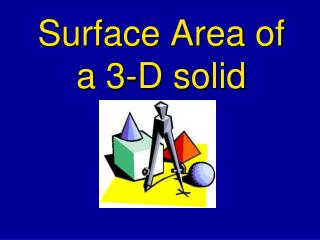# Surface Area of a 3-D solid - PowerPoint PPT PresentationDownload PresentationSurface Area of a 3-D solid

Surface Area of a 3-D solidDownload Presentation## Surface Area of a 3-D solid

- - - - - - - - - - - - - - - - - - - - - - - - - - - E N D - - - - - - - - - - - - - - - - - - - - - - - - - - -
##### Presentation Transcript

1. Surface Area of a 3-D solid

2. Definition • Surface Area – is the total number of unit squares used to cover a 3-D surface.

3. Find the SA of a Rectangular Solid A rectangular solid has 6 faces. They are: Front • Top • Bottom • Front • Back • Right Side • Left Side Top Right Side We can only see 3 faces at any one time. Which of the 6 sides are the same? • Top and Bottom • Front and Back • Right Side and Left Side

4. Surface Area of a Rectangular Solid We know that Each face is a rectangle. and the Formula for finding the area of a rectangle is: A = lw Front Top Right Side Steps: Find: Area of Top Area of Front Area of Right Side Find the sum of the areas Multiply the sum by 2. The answer you get is the surface area of the rectangular solid.

5. Find the Surface Area of the following: Find the Area of each face: 12 m Top 5 m A = 12 m x 5 m = 60 m2 Top Front Right Side 8 m Front 8 m A = 12 m x 8 m = 96 m2 5 m 12 m 12 m Right Side Sum = 60 m2 + 96 m2 + 40 m2 = 196 m2 A = 8 m x 5 m = 40 m2 8 m Multiply sum by 2 = 196 m2 x 2 = 392 m2 5 m The surface area = 392 m2

6. Find the Surface Area Area of Top = 6 cm x 4 cm = 24 cm2 Area of Front = 14 cm x 6 cm = 84 cm2 24 m2 Area of Right Side = 14 cm x 4 cm = 56 cm2 Find the sum of the areas: 56 m2 84 m2 14 cm 24 cm2 + 84 cm2 + 56 cm2 = 164 cm2 Multiply the sum by 2: 4 cm 164 cm2 x 2 = 328 cm2 6 cm The surface area of this rectangular solid is 328 cm2.

7. Nets • A net is all the surfaces of a rectangular solid laid out flat. Back 8 cm Top Left Side Top Right Side 5 cm 8 cm Right Side Front 8 cm Front 8 cm 5 cm 10 cm Bottom 5 cm 10 cm

8. Find the Surface Area using nets. Back Top 8 cm 80 Right Side Front 40 Top 40 8 cm 50 Right Side 5 cm Left Side 5 cm 8 cm 10 cm Front 8 cm 80 Each surface is a rectangle. A = lw Bottom 5 cm 50 Find the area of each surface. Which surfaces are the same? Find the Total Surface Area. 10 cm What is the Surface Area of the Rectangular solid? 340 cm2

9. Find the Surface Area using a Formula S.A. = 2(area of the base) + (perimeter of the base) • height S.A. = 2(2ft. X 3ft.) + (2ft + 3ft + 2ft + 3ft) x 6ft Answer: 72ft²

10. Surface Area of a Pyramid S.A. = Area of the base + 4(area of the triangular face) S.A. = 7ft x 7ft + 4(1/2 x 7ft x 8.7ft) S.A. = 49ft² + 121.8ft² = 170.8ft²

11. Surface Area of a Cylinder S.A. = 2(Area of the base) + Circumference of the base x height of the cylinder S.A. = 2(3.14 x 10m²) + 20m x 3.14 x 13m S.A. = 628m² + 816.4m² = 1444.4m²

12. Now let’s do some practice problems! Pg. 568 #1-6 & Pg. 571 #1-3